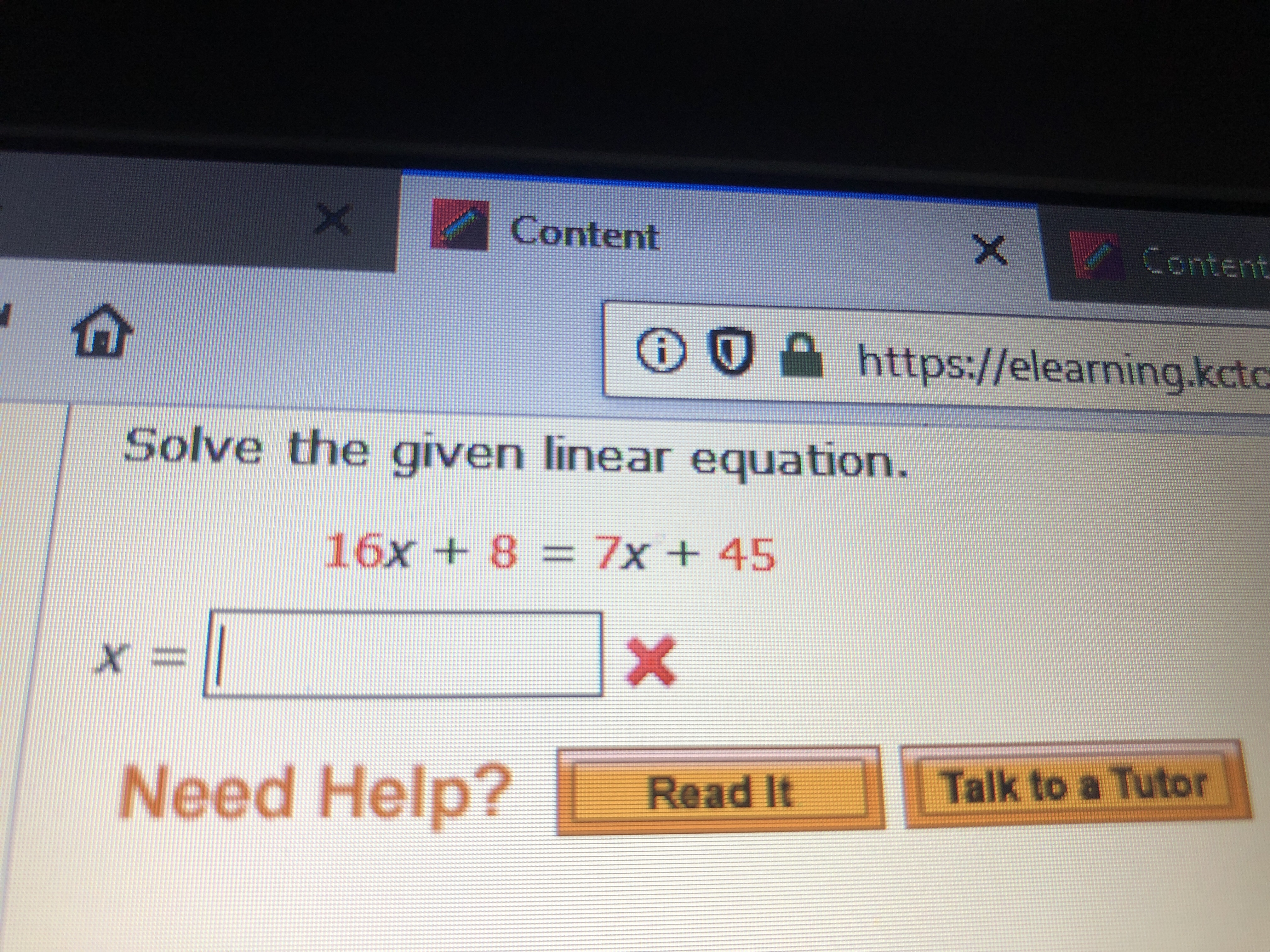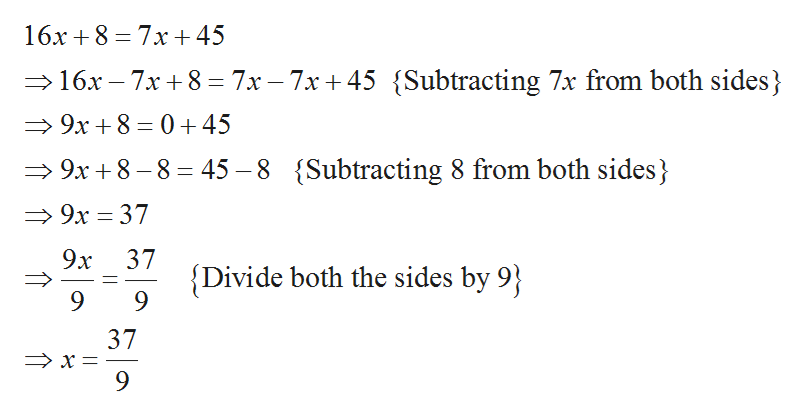# X ContentContenthttps://elearning.kctcSolve the given linear equation.16x +8 = 7x + 45XNeed Help?Talk to a TutorRead It

Question
3 views

what is the blankhelp_outlineImage TranscriptioncloseX Content Content https://elearning.kctc Solve the given linear equation. 16x +8 = 7x + 45 X Need Help? Talk to a Tutor Read It fullscreen
check_circle

Step 1

It is required to solve the linear equation for the variable x, the linear equation is given below,

Step 2

The calculation for the equation 16x + 8 = 7x...help_outlineImage Transcriptionclose16x 8 7x45 16x-7x8 7x-7x+ 45 Subtracting 7x from both sides 9x 8 045 9x +8-8 45-8 {Subtracting 8 from both sides 9х — 37 9х Divide both the sides by 9 37 9 fullscreen

### Want to see the full answer?

See Solution

#### Want to see this answer and more?

Solutions are written by subject experts who are available 24/7. Questions are typically answered within 1 hour.*

See Solution
*Response times may vary by subject and question.
Tagged in

### Equations and In-equations